Algebra 2 4-1 Complete Lesson: Quadratic Functions and Transformations
starstarstarstarstarstarstarstarstarstar
4.5 (2 ratings)
by Matthew Richardson
| 25 Questions
Note from the author:
A complete formative lesson with embedded slideshow, mini lecture screencasts, checks for understanding, practice items, mixed review, and reflection. I create these assignments to supplement each lesson of Pearson's Common Core Edition Algebra 1, Algebra 2, and Geometry courses. See also mathquest.net and twitter.com/mathquestEDU.The outlined content above was added from outside of Formative.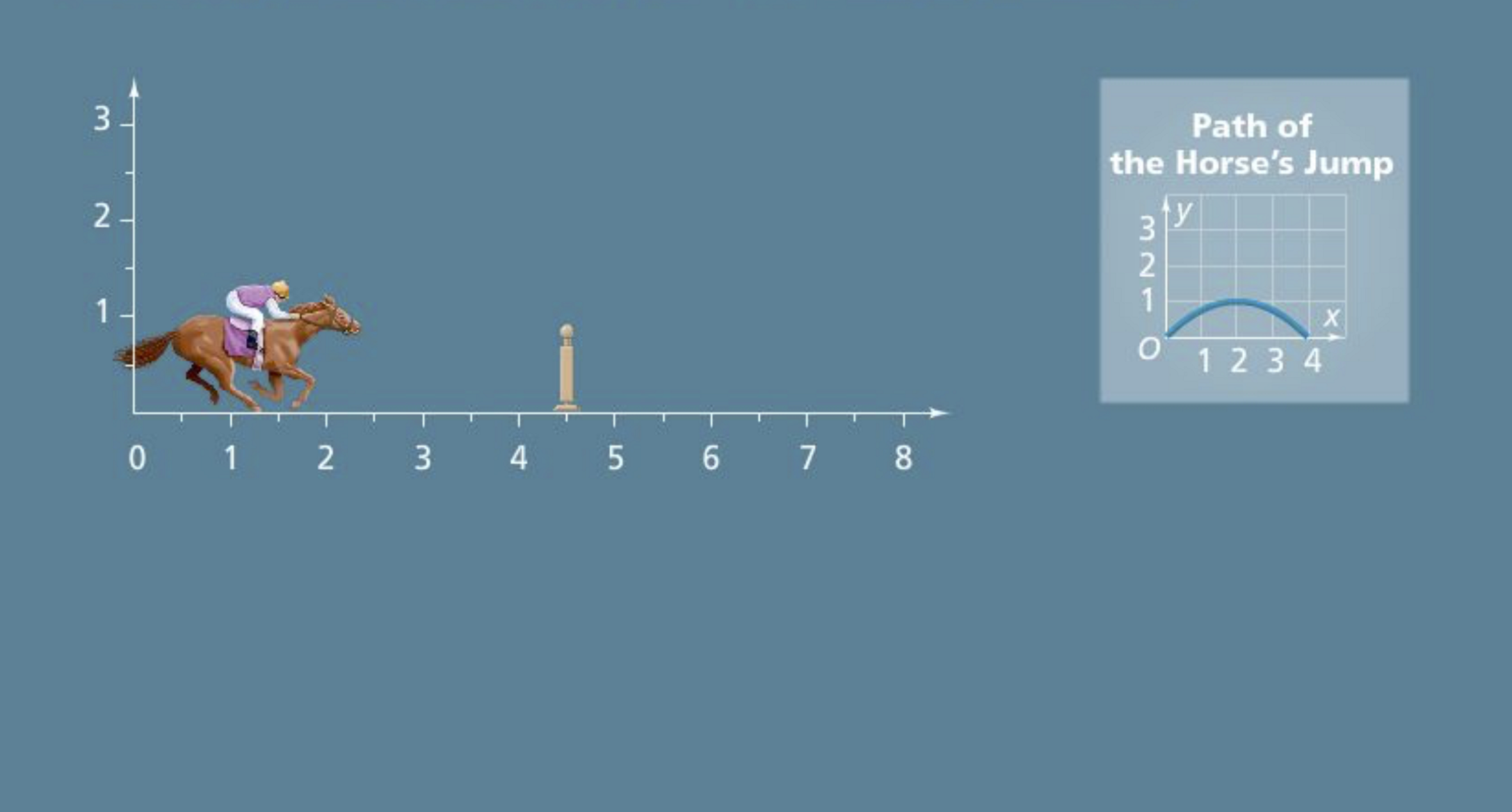a
1
Solve It! In the computer game Steeplechase, you press the "jump" button and the horse makes the jump shown. The highest part of the jump must be directly above the fence or you lose time.
1
10 pts
Solve It! Where should this horse be when you press "jump"?
at 4 on the horizontal axis
at 2.5 on the horizontal axis
at 3.5 on the horizontal axis
at 3 on the horizontal axis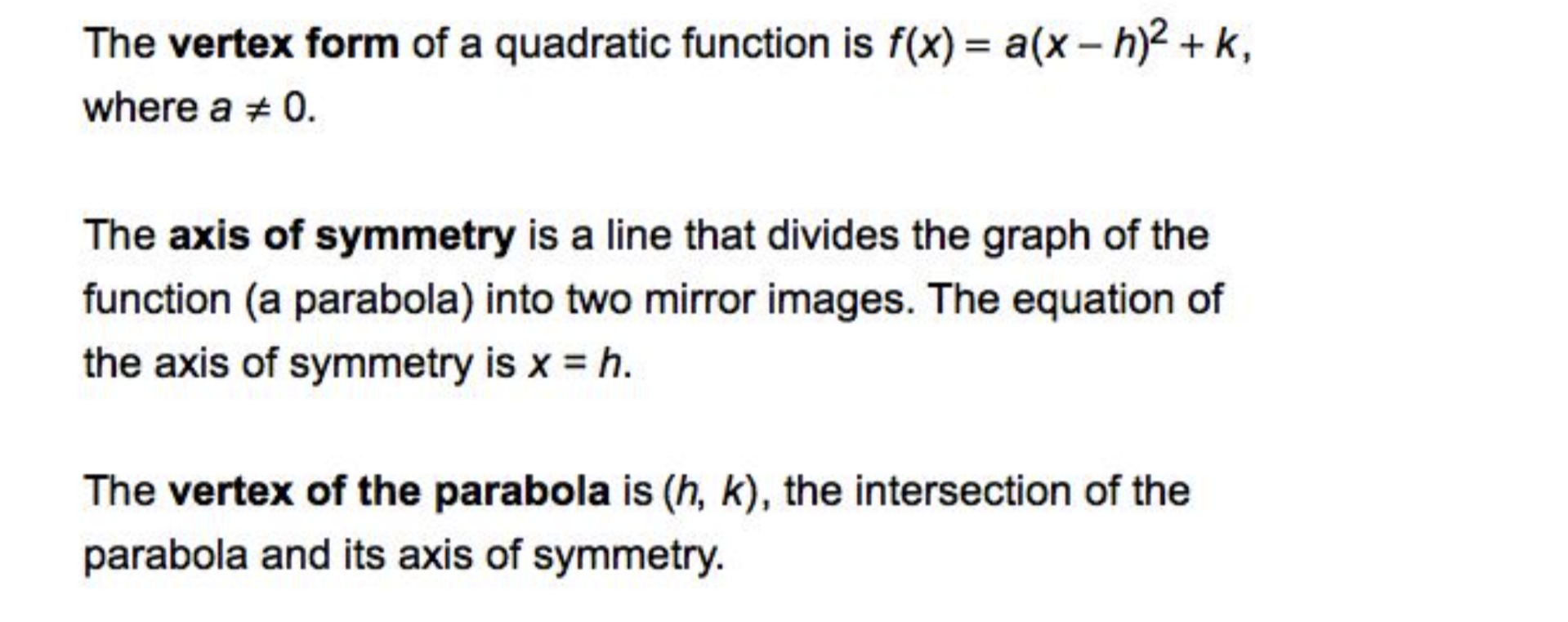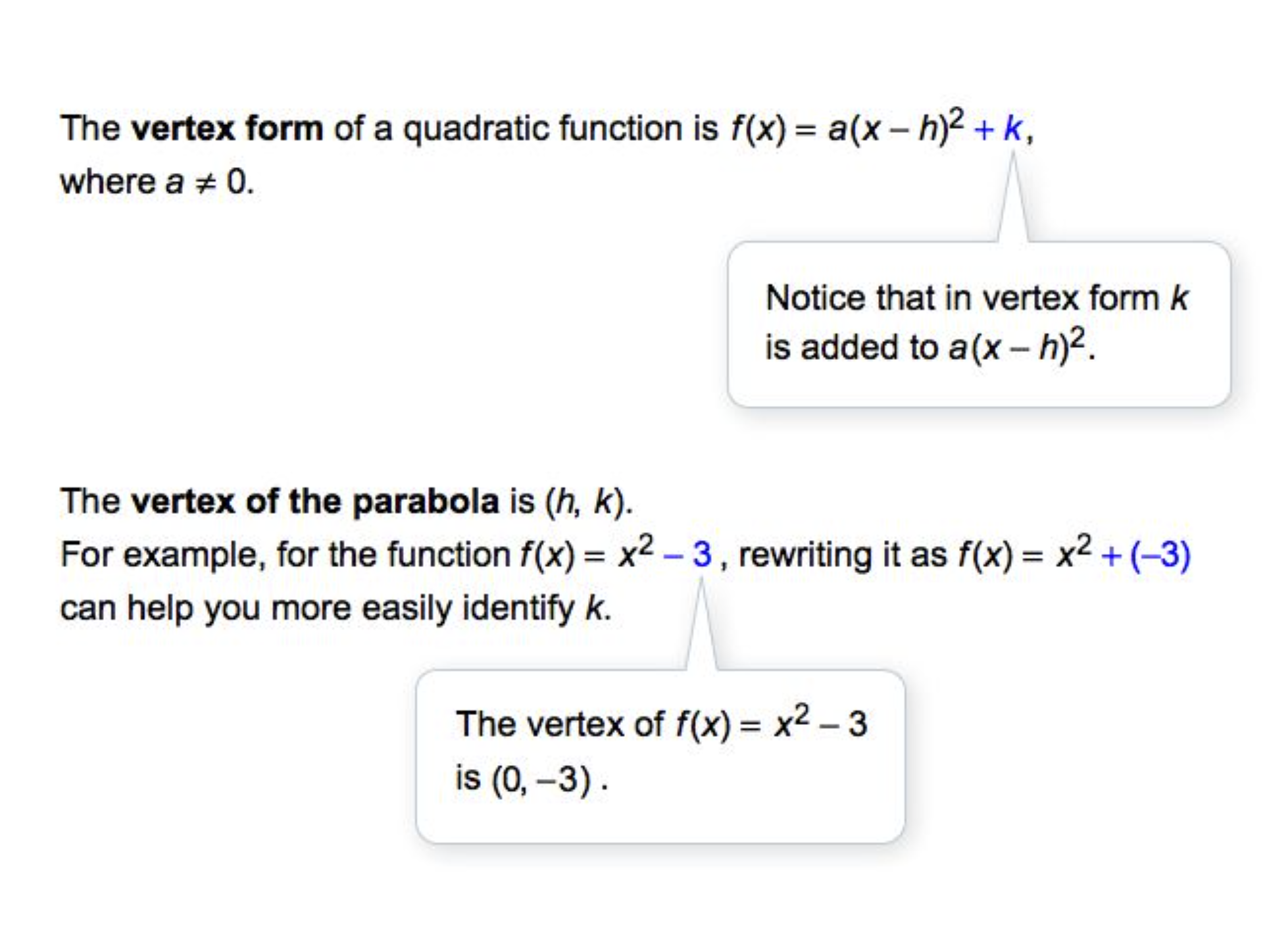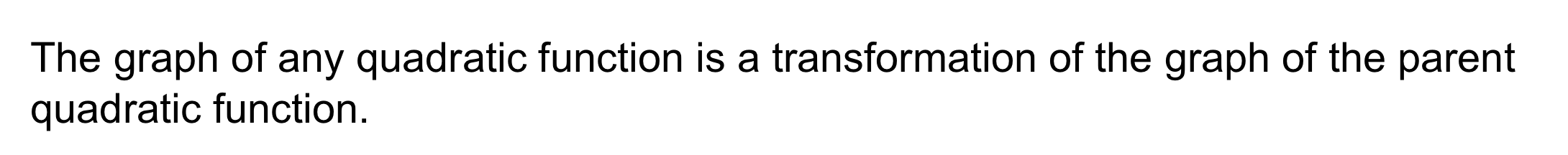2
20 pts
Problem 1 Got It? What is the graph of the function? Complete the table of values and graph the function on the canvas. Use colors other than black.

3
10 pts
Problem 1 Got It? Graph the parent quadratic function and its transformation on the same plane. Zoom and pan your graph to establish an appropriate viewing window. Consider the relationship between the two functions.
After graphing with the Desmos utility, you may edit your response to the previous item if needed.
4
10 pts
Problem 1 Got It? Reasoning: What can you say about the graph of the function below if a is a negative number?

The graph is a parabola that opens downward.
The graph is a parabola that opens upward.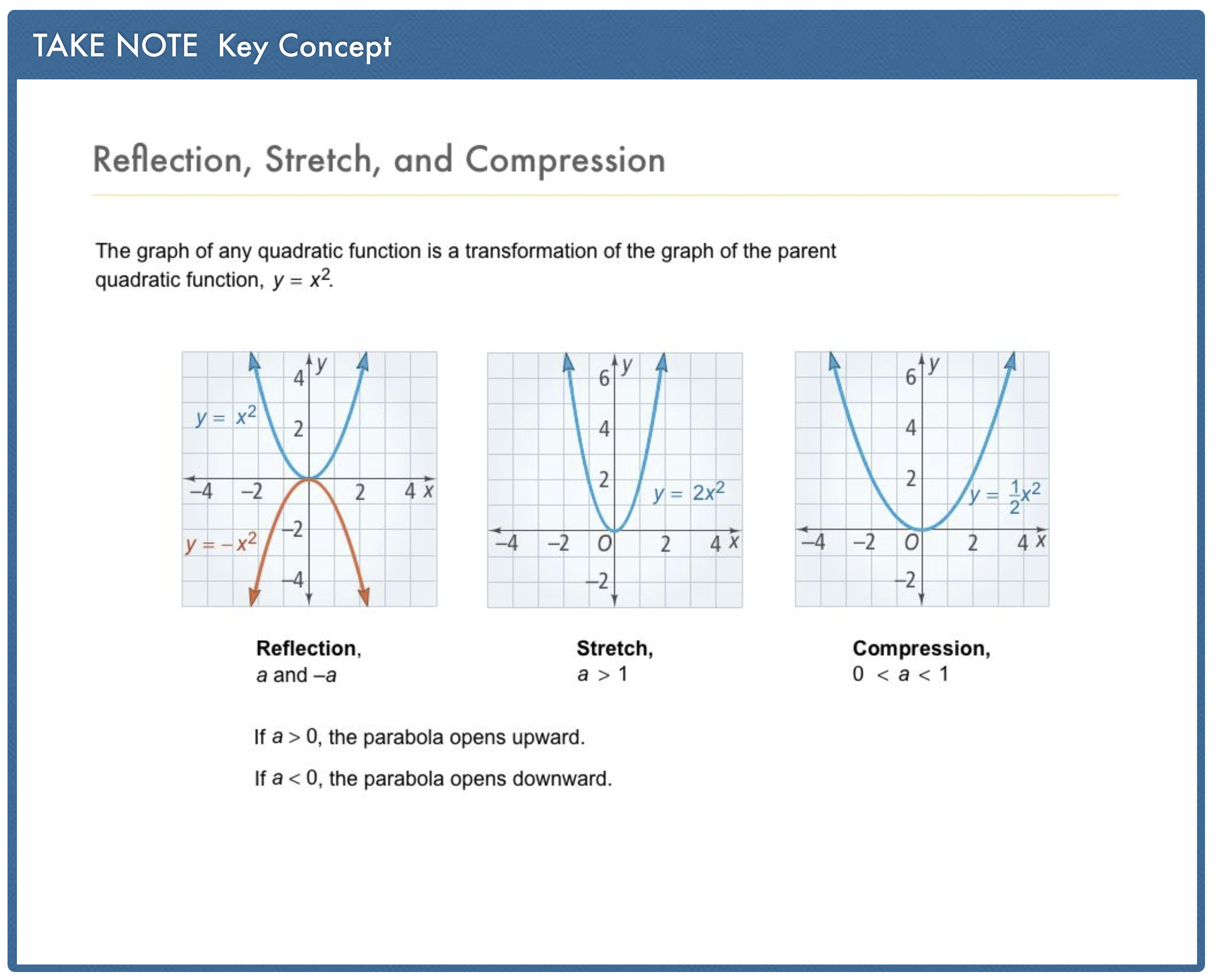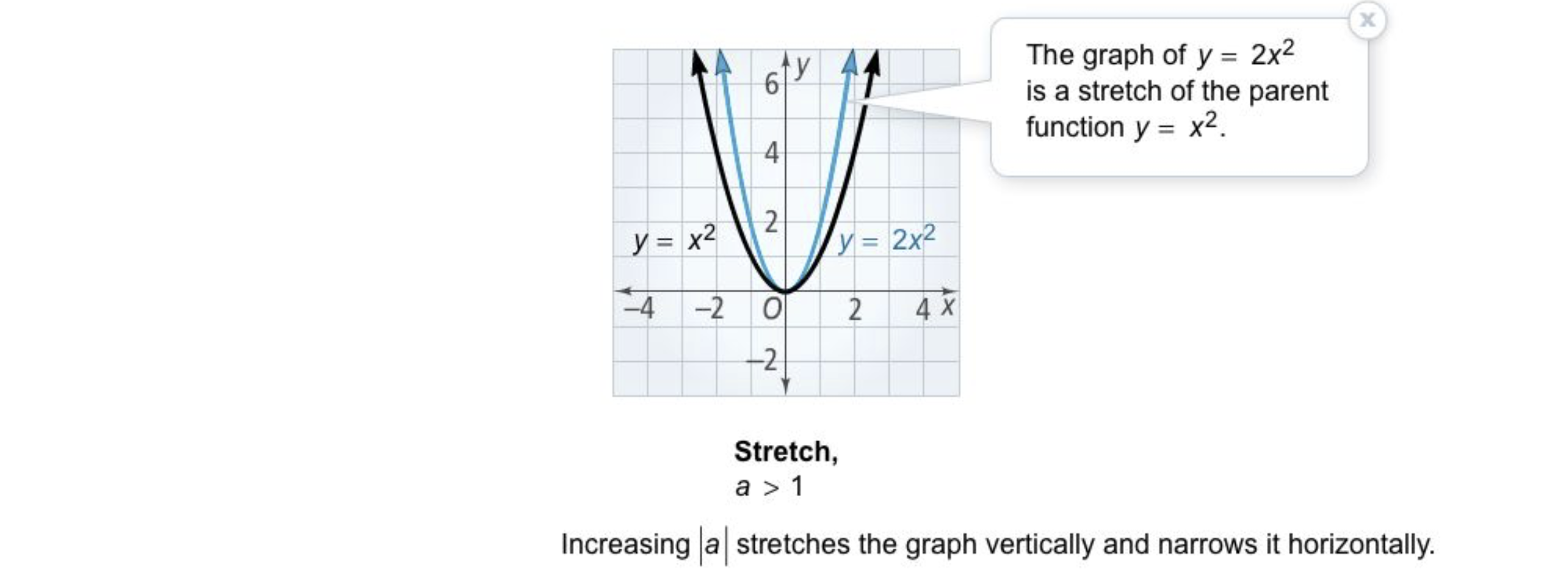5
20 pts
Problem 2 Got It? Sketch a graph the function on the canvas. Include relevant graph detail: label axes, indicate units and scale on both axes, and use arrows to represent end behavior as appropriate.

6
10 pts
Problem 2 Got It? How is the graph of g(x) = + 3 in the previous item a translation of the parent function f(x) = ?
g(x) is f(x) translated up 3 units
g(x) is f(x) translated left 3 units
g(x) is f(x) translated down 3 units
g(x) is f(x) translated right 3 units
7
20 pts
Problem 2 Got It? Sketch a graph the function on the canvas. Include relevant graph detail: label axes, indicate units and scale on both axes, and use arrows to represent end behavior as appropriate.

8
10 pts
Problem 2 Got It? How is the graph of h(x) = (x + 1)² in the previous item a translation of the parent function f(x) = ?
h(x) is f(x) translated right 1 unit
h(x) is f(x) translated down 1 unit
h(x) is f(x) translated left 1 unit
h(x) is f(x) translated up 1 unit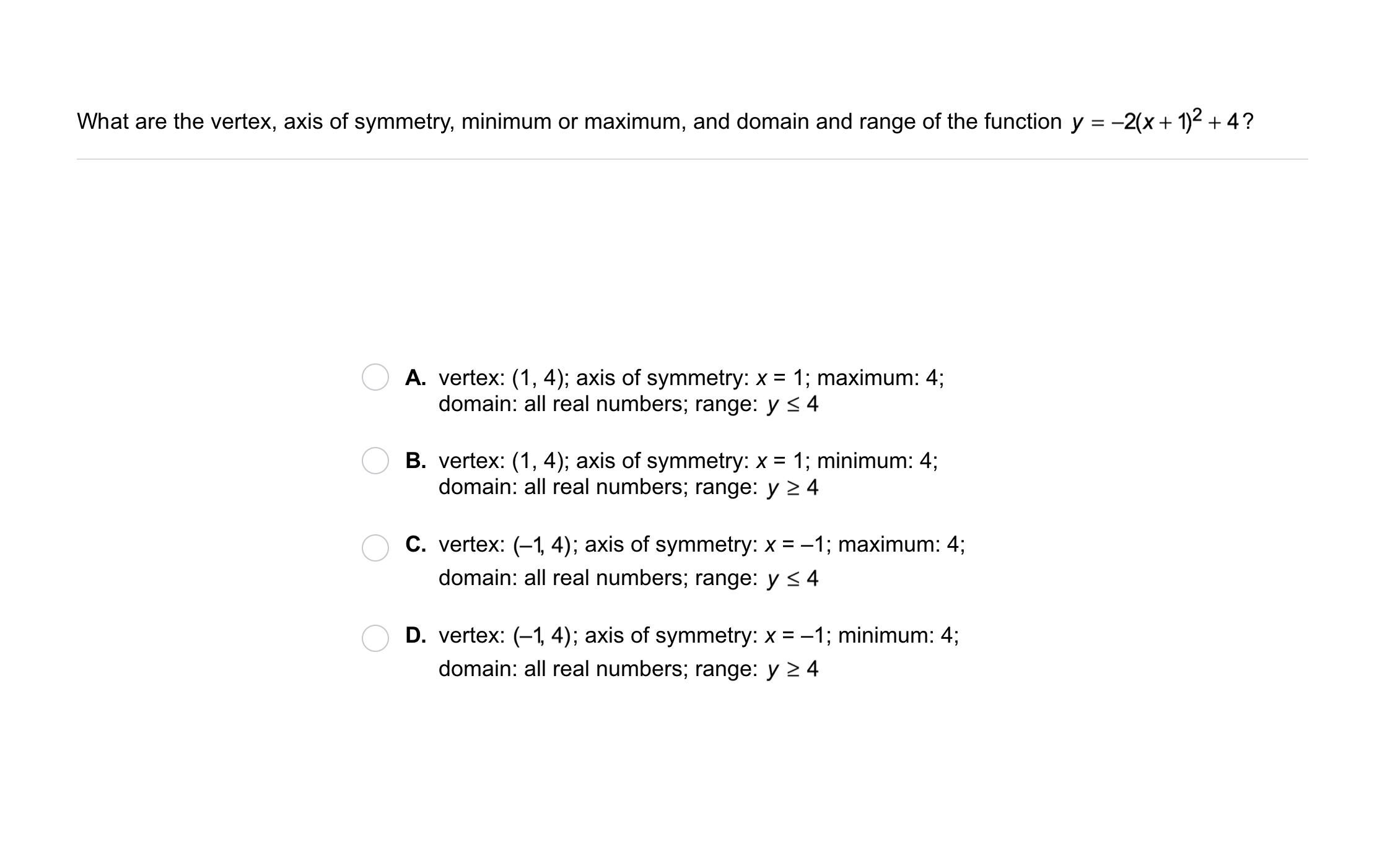9
9
10 pts
Problem 3 Got It?
A
B
C
D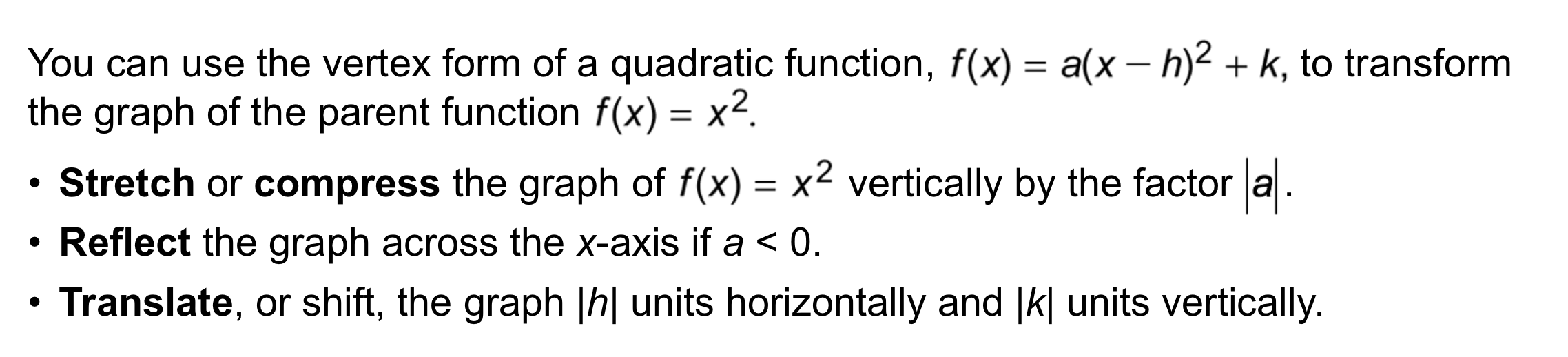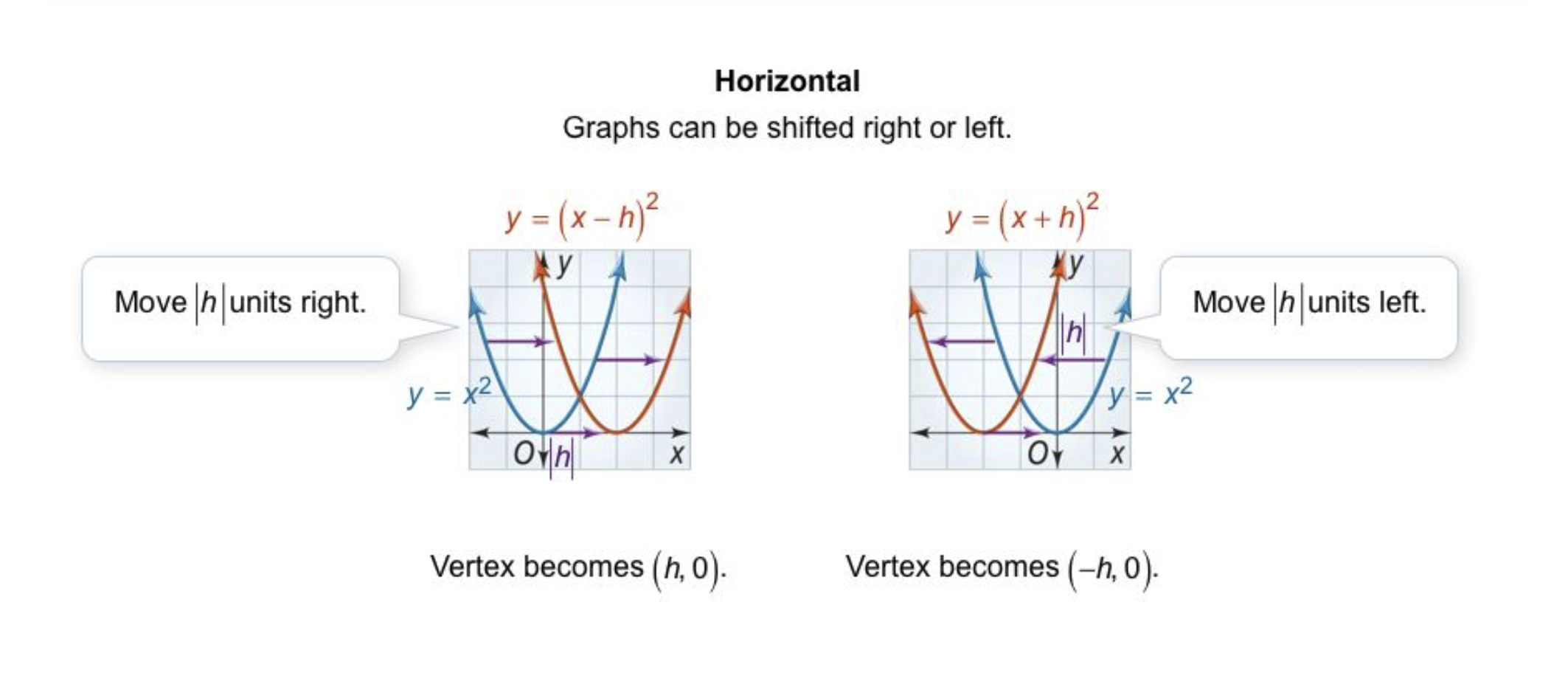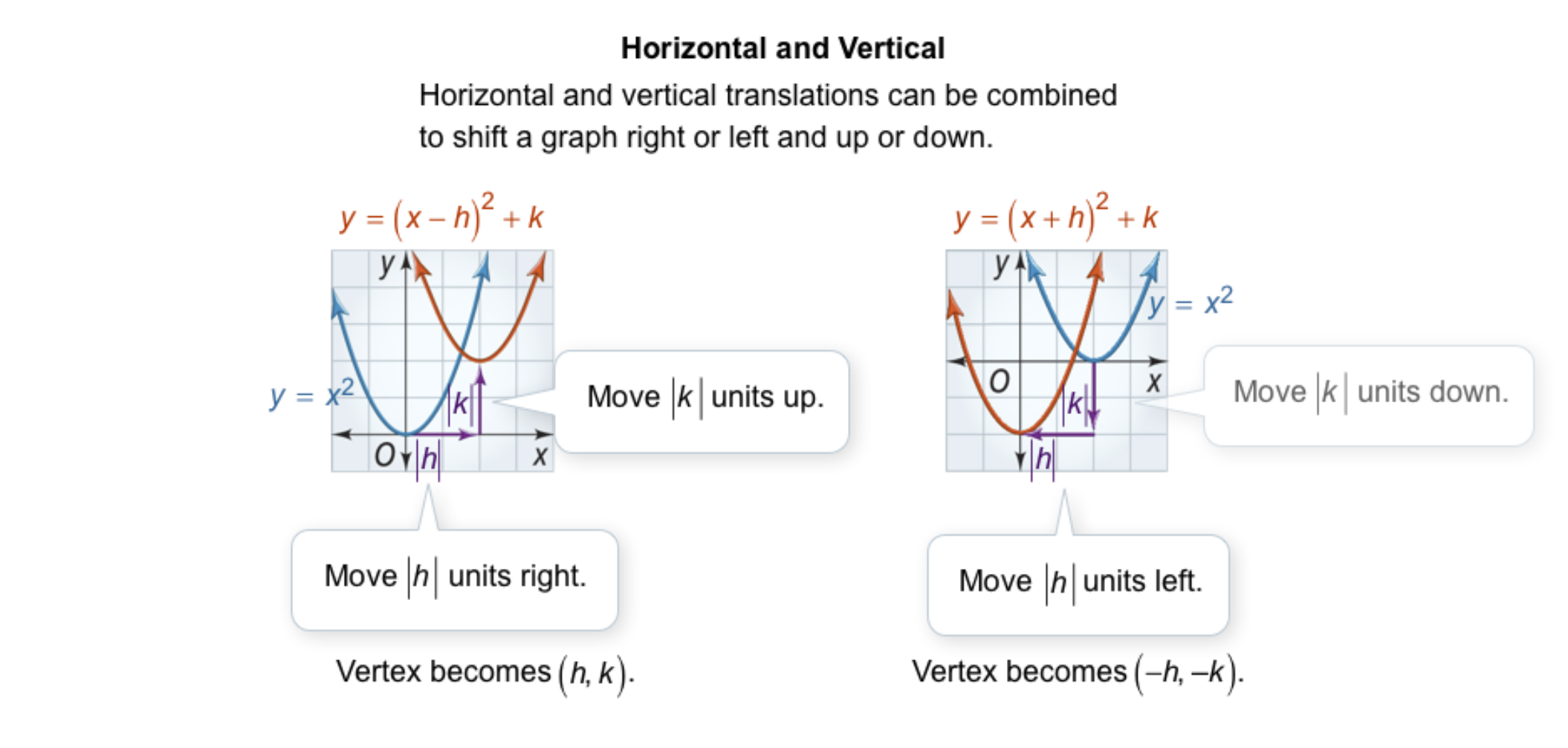10
10
10 pts
Problem 4 Got It?
A
B
C
D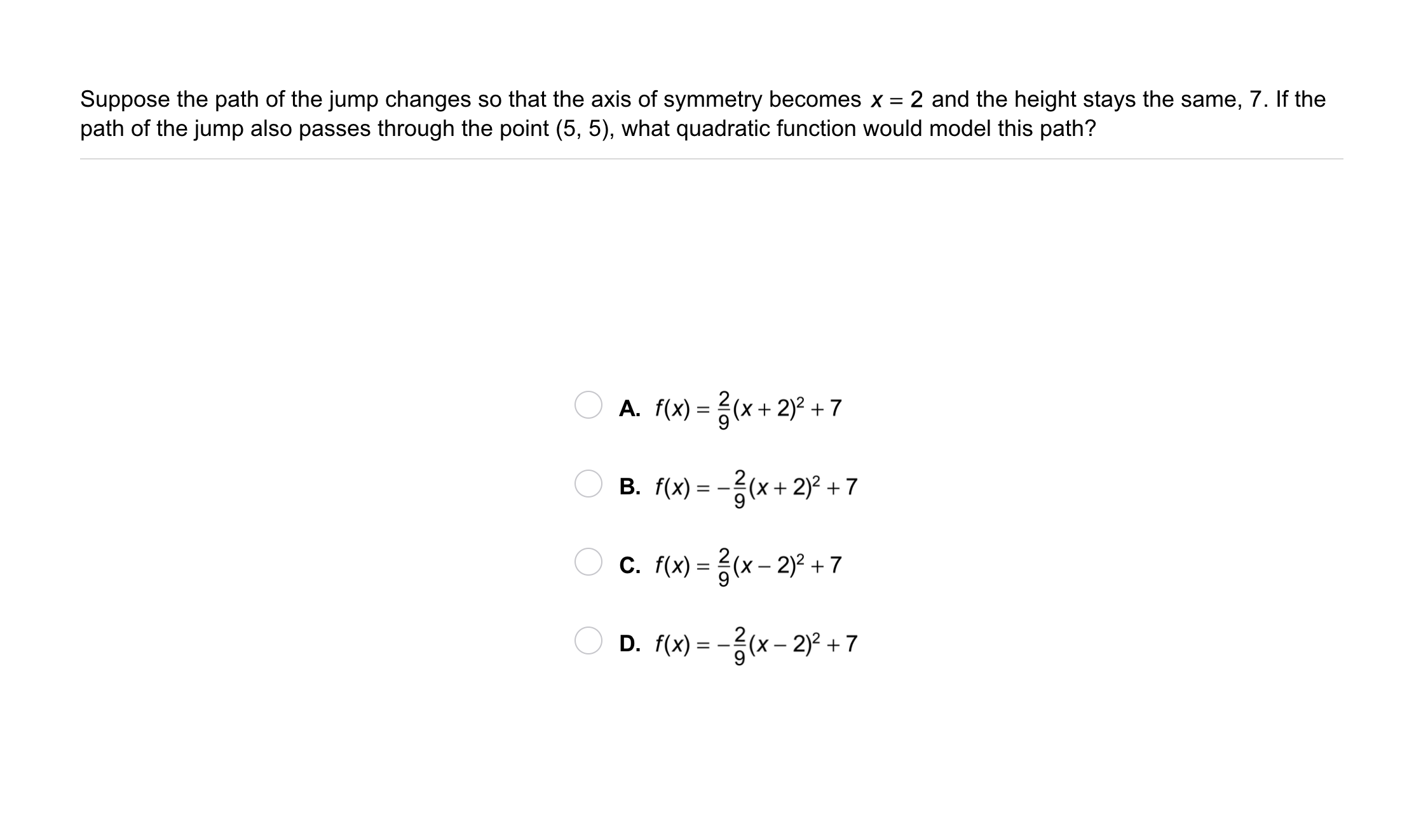11
11
10 pts
Problem 5 Got It? Suppose the path of the jump changes so that the axis of symmetry becomes x = 2 and the height stays the same, 7. If the path of the jump also passes through the point (5, 5), what quaratic function would model this path?
A
B
C
D12
20 pts
Graphing: Sketch a graph of the function on the canvas. Include relevant graph details.

The outlined content above was added from outside of Formative.
13
10 pts
Analysis: Determine whether the function has a maximum or a minimum value. First complete without using graphing, but you may check your response on the embedded Desmos calculator.

minimum (upward-opening parabola)
maximum (downward-opening parabola)
14
10 pts
Conversion: Rewrite the equation in vertex form.16
10 pts
Reasoning: Is the equation a quadratic function? Explain.

No; Since (x - 4) squared is multiplied by 0, the function is reduced to y = 3. It is linear, not quadratic.
Yes; Since (x -4) is squared, the function is a quadratic.
17
10 pts
Compare and Contrast: Describe the differences between the graphs of these functions.18
10 pts
Review Lesson 3-6: Solve the system of equations using a matrix. Show your steps (row operations) and identify the solution on the canvas. You may use rectangles as matrix frames for convenience. The first frame is created for you.

19
10 pts
Review Lesson 4-2: Graph the following absolute value functions on the same plane. Zoom and pan your graph to establish an appropriate viewing window.

20
10 pts
Review Lesson 4-2: Match the absolute value function with its vertex.

• (5, 0)
• (0, 0)
• (-1, 0)21
10 pts
Vocabulary Review: Circle the vertex of each absolute value graph. Use contrasting colors.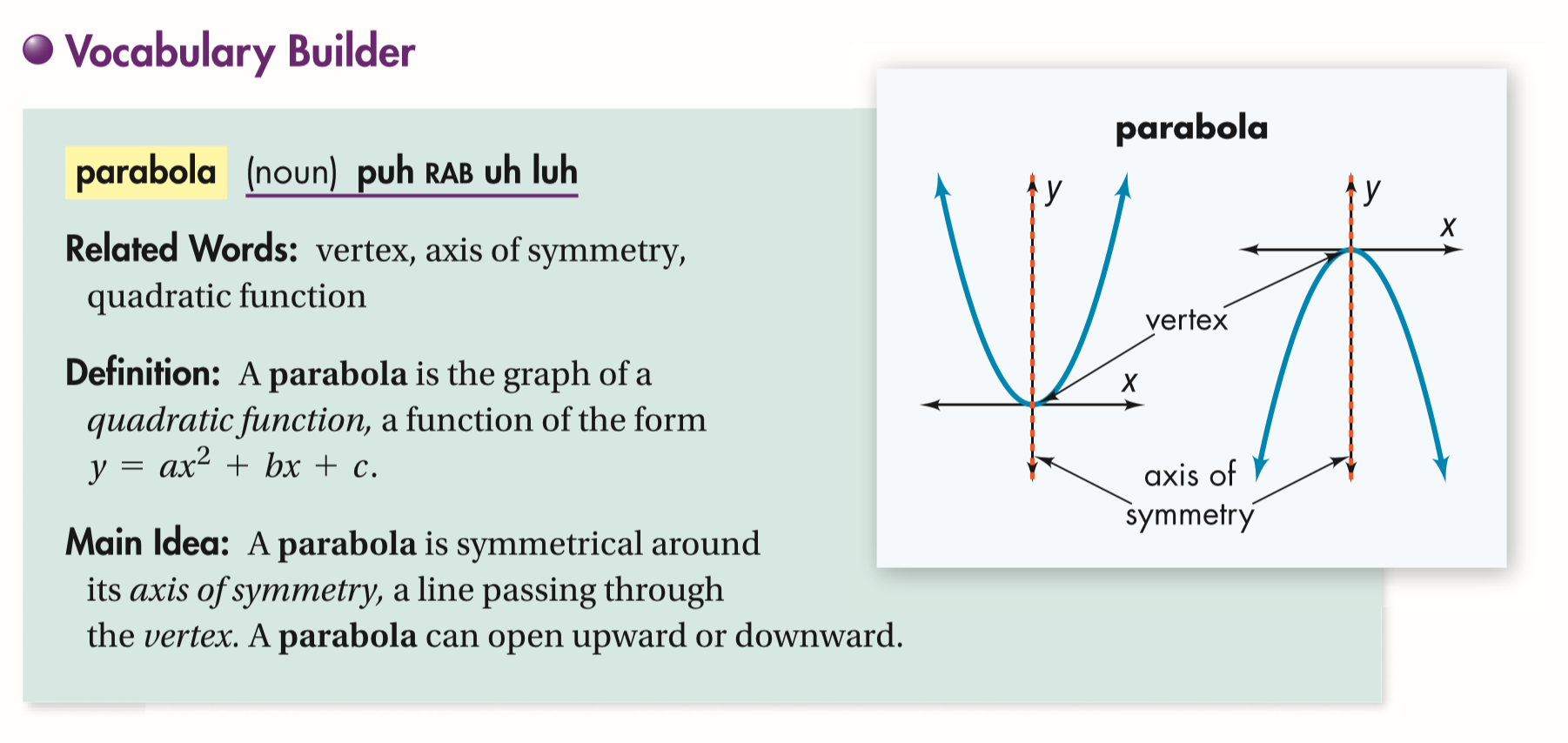22
10 pts
Use Your Vocabulary: Categorize each function based on whether or not its graph is a parabola.

• Graph is a parablola
• Graph is NOT a parabola
23
10 pts
Translations: Match each parabola with its function. Assume that each grid line represents 1 unit.

• y = x² - 1
• y = (x + 1)² - 2
• y = (x - 2)² + 3
24
100 pts
Notes: Take a clear picture or screenshot of your Cornell notes for this lesson. Upload it to the canvas. Zoom and pan as needed.

For a refresher on the Cornell note-taking system, click here.
25
10 pts
Reflection: Math Success
Add to my formatives list

Formative uses cookies to allow us to better understand how the site is used. By continuing to use this site, you consent to the Terms of Service and Privacy Policy.